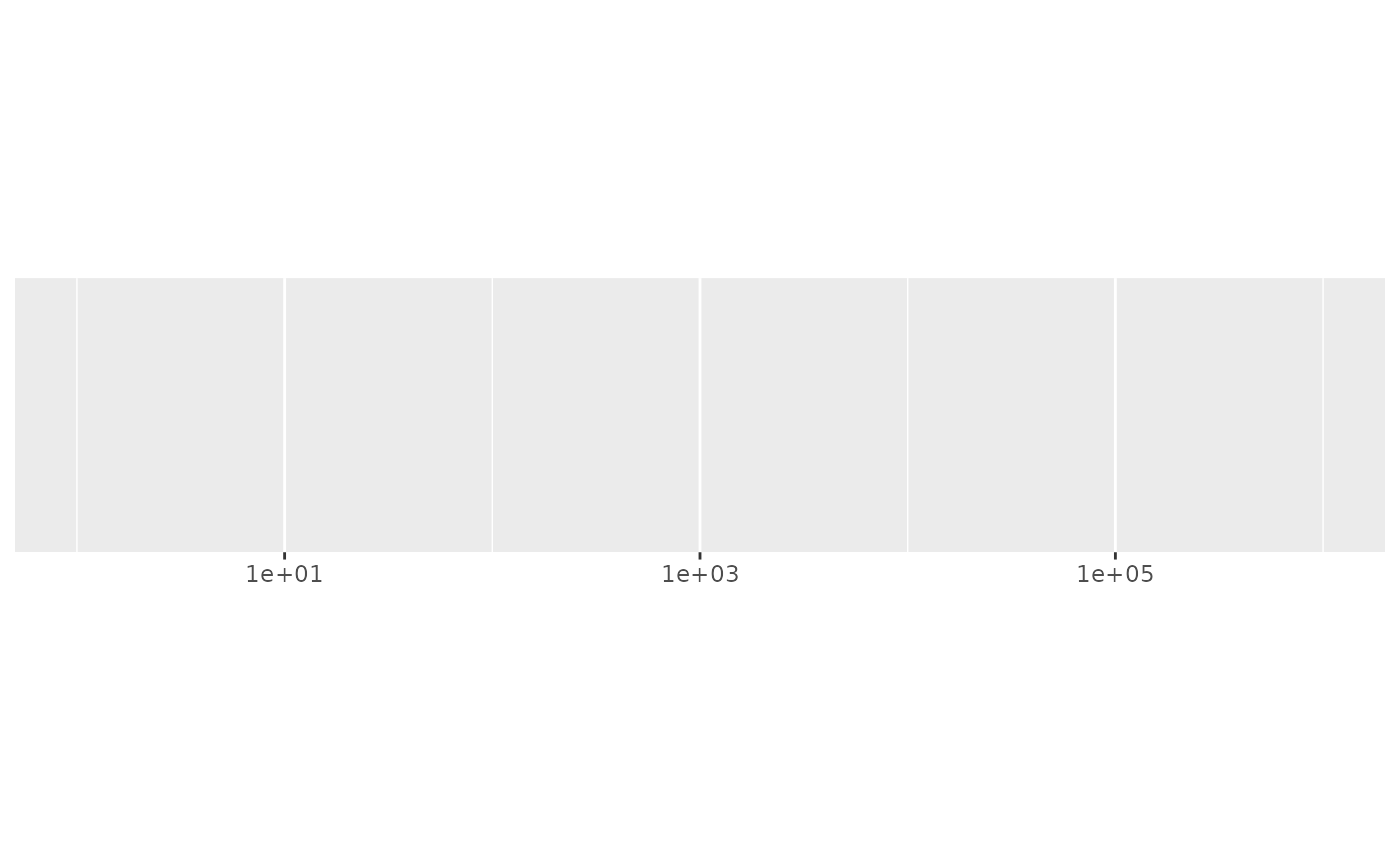Generate minor breaks between major breaks either spaced with a fixed width, or having a fixed number.

## Usage

minor_breaks_width(width, offset)

minor_breaks_n(n)

## Arguments

width

Distance between each break. Either a number, or for date/times, a single string of the form "{n} {unit}", e.g. "1 month", "5 days". Unit can be of one "sec", "min", "hour", "day", "week", "month", "year".

offset

Use if you don't want breaks to start at zero, or on a conventional date or time boundary such as the 1st of January or midnight. Either a number, or for date/times, a single string of the form "{n} {unit}", as for width.

offset can be a vector, which will accumulate in the order given. This is mostly useful for dates, where e.g. c("3 months", "5 days") will offset by three months and five days, which is useful for the UK tax year. Note that due to way that dates are rounded, there's no guarantee that offset = c(x, y) will give the same result as offset = c(y, x).

n

number of breaks

## Examples

demo_log10(c(1, 1e6))
#> scale_x_log10()if (FALSE) {
# Requires https://github.com/tidyverse/ggplot2/pull/3591
demo_log10(c(1, 1e6), minor_breaks = minor_breaks_n(10))
}